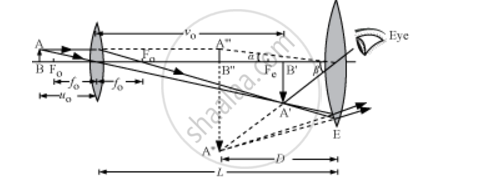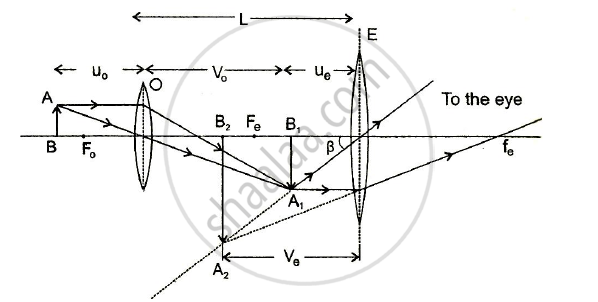# Draw a Labelled Ray Diagram Showing the Formation of a Final Image by a Compound Microscope at Least Distance of Distinct Vision - Physics (Theory)

Draw a labelled ray diagram showing the formation of a final image by a compound microscope at least distance of distinct vision

Draw a ray diagram for the formation of image by a compound microscope

#### Solution 1where, AB = object, A'B' = image formed by objective and A''B'' = image formed by eyepiece

fo = focal length of objective,

uo = object distance from objective

vo = image distance from objective

D = distance of least distinct vision

L = length of the microscope

#### Solution 2Concept: Optical Instruments - The Microscope
Is there an error in this question or solution?